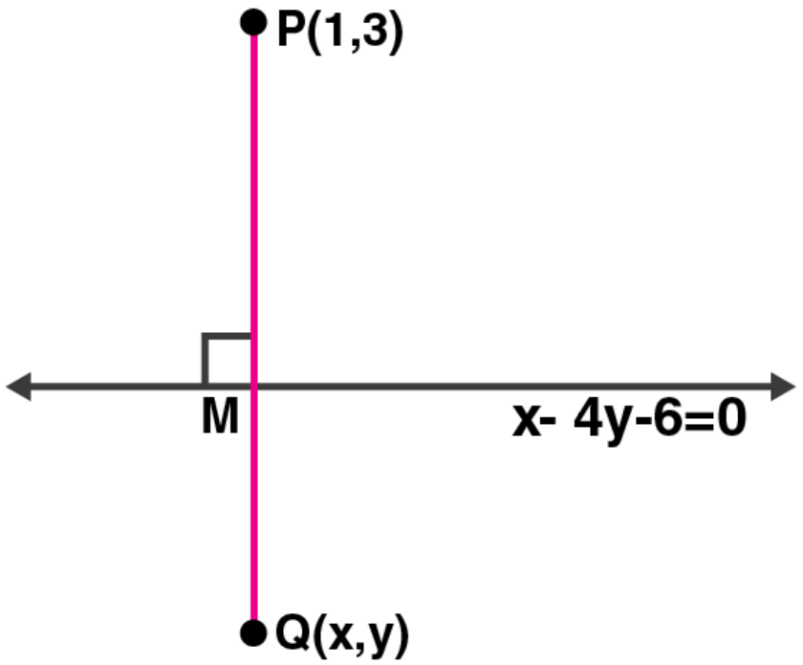Newbie

# If the line x – 4y – 6 = 0 is the perpendicular bisector of the line segment PQ and the co-ordinates of P are (1, 3), find the co-ordinates of Q.

• 1

This question is Based on Equation of a Straight Line Chapter of M.L Aggarwal book for ICSE BOARD for class 10.
Here if a line is the perpendicular bisector of a line segment. and the co-ordinates of P are (1, 3), find the co-ordinates of Q.
This is the Question Number 41, Exercise 12.2 of M.L Aggarwal.

Share

1.Given, line equation: x – 4y – 6 = 0 … (i)

Co-ordinates of P are (1, 3)

Let the co-ordinates of Q be (x , y)

Now, the slope of the given line is

4y = x – 6

y = (1/4) x – 6/4

slope (m) = ¼

So, the slope of PQ will be (-1/m) [As the product of slopes of perpendicular lines is -1]

Slope of PQ = -1/ (1/4) = -4

Now, the equation of line PQ will be

y – 3 = (-4) (x – 1)

y – 3 = -4x + 4

4x + y = 7 … (ii)

On solving equations (i) and (ii), we get the coordinates of MMultiplying (ii) by 4 and adding with (i), we get

x – 4y – 6 = 0

16x + 4y = 28

——————

17x = 34

x = 34/17 = 2

Putting the value of x in (i)

2 – 4y – 6 = 0

-4 – 4y = 0

4y = -4

y = -1

So, the co-ordinates of M are (2, -1)

But, M is the mid-point of line segment PQ

(2, -1) = (x + 1)/2 , (y + 3)/2

(x + 1)/2 = 2

x + 1 = 4

x = 3

And,

(y + 3)/2 = -1

y + 3 = -2

y = -5

Thus, the co-ordinates of Q are (3, -5).

• 2